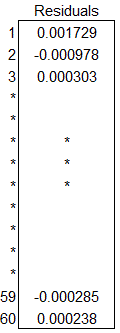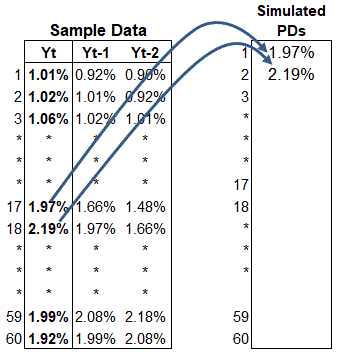# How to quantify Model Risk from Parameter Uncertainty

For a quantitative analyst whose models are frequently scrutinized by Federal Reserve Bank examiners, the ability to quantify model risk is an important part of the model documentation process. Model risk is typically described as “. . . the potential for adverse consequences from decisions based on incorrect or misused model outputs and reports.”

Model Risk quantification can be a tricky concept to grasp.  But when we consider that models are nothing more than abstractions of real life situations, it’s easier to see how there are risks associated with models. Even when models perform exceptionally well in recreating said real life scenario.A typical strategy for building a model proceeds as follows. A model builder uses some theory or intuition to develop a model. The model is then implemented, usually by a programmer or potentially the model builder him/herself. Finally, the model is put into use for the purpose it was developed.

Each of these three steps poses its own unique risk: (a) model specification errors can arise, for example, by incorrectly applying the underlying model theory, (b) model implementation errors are generally fairly common and result from coding errors or bugs in programs, and (c) human error and parameter measurement error, or parameter uncertainty, are introduced during the actual use of the model. To demonstrate parameter uncertainty, the default rate for a particular risk bucket used in a credit VaR (CVaR) model can be estimated to be 0.25% when it’s actually closer to 1%. This biased value can lead to gross underestimation of the final cVaR estimate.The 2007 financial crisis is an example of how models when left unchecked can have catastrophic results. Pre-2007, the historical data used to calibrate many mortgage models painted quite a rosy picture of  housing prices, one where housing prices were projected to continually rise.  The crash in housing prices that was experienced during 2007-2009 was not considered in the models, so those models were not correctly calibrated to account for the actual levels of unexpected (tail) risk reached during that period.

During subsequent years, through the urging of Federal regulators and government legislation via the Dodd-Frank Consumer Protection Act, financial institutions have had to beef up their internal Model governance resources as they are defined as the first line of defense for monitoring Model Risk. These are independent teams with enterprise-wide mandates to help ensure the soundness of models, help identify limitations, ensure proper documentation, and other oversight issues. Gone are the days when model developers could blithely dismiss the suggestions and issues raised by internal governance teams. Action plans and serious remediation efforts by developers are commonplace in the current environment, and executive leadership routinely views these activities as high corporate priorities.

Sources of model risk

In practice, financial models vary greatly in terms of complexity and structure. As a consequence, measuring model risk through quantitative means can be very challenging as it must account for a financial entity’s unique characteristics. As indicated above, financial model risk typically arises from four main sources:

• Model specification error – This type of risk may arise from omitting key risk factors or using incorrect assumptions about the shape of a distribution. An obvious example is using  OLS framework,$Y_i = \beta_0 + \beta_1 X_1 + \beta_2 X_2 + \epsilon_i$

where it is more appropriate to apply a Logistic regression model,$\ln(\frac {p_i}{1-{p_i}}) = \beta_0 + \beta_1 X_1 + \beta_2 X_2 + ... + \beta_k X_k$

• Parameter uncertainty – This type of risk always exists, since stochastic models assume that unknown parameters may be estimated, but are rarely perfectly accurate. There is always some uncertainty associated with estimating a particular model. For example, using through-the-cycle, long run average PDs (estimated with data from only expansionary time periods) in building a model to project economic adversity will certainly propagate some variability. Parameter uncertainty can come in two flavors:
• Variability due to parameter estimation
• Insufficient or incorrect data
• Faulty implementation – Poor coding design, incorrect algorithms, and bugs can lead to spurious results.
• User error – If, for example, multiple users of the same model are asked to recalculate an outcome, the expectation is that the model will produce the same results based on the inputs. User error can result if other users are unable to replicate a given set of output with the same inputs used by the developer. With complicated models lacking sufficient documentation and/or training manuals, user errors are much more likely.Model Risk for Credit Risk Models

Model implementation and user errors do not permit themselves being easily measured. Often, financial institutions resort to simplifying measures with the help of model risk scorecards and expert judgment.

The quantification of model specification error can be approached from two perspectives: (1) taking some sort of average of the available competing models, and (2) benchmarking against industry standard models. In these scenarios, the more models you have, the more reliable your Model Risk estimate can be. This is where consulting agencies can add a considerable amount of value.  They have the luxury of having access to multiple model approaches across the industry, and are thus in the position of making these types of comparisons. It is worthwhile to note that even the reference industry models themselves can have specification error built in, which emphasizes the importance of conservatism in model development.

Model Risk using a VaR framework

A straightforward method to internally quantify Model Risk, in the context of portfolio-level Credit Risk modeling, is through parameter uncertainty (in effect, taking into account parameter estimation error due to the impact of noisy inputs used in the models). We consider the VaR model approach to illustrate this technique.

Credit VaR distributions accept multiple factors, or parameters, in the construction, describing their shape and position. A naïve-CVaR model uses factors that do not reflect that uncertainty related to estimating that input to the CVaR model. This factor can be represented as a point estimate, like a simple average.

To develop a more conservative approach, the measure of uncertainty can be used to wholly adjust the model parameters themselves, and consequently the CVaR distribution and final VaR estimate, as follows. The uncertainty associated with each parameter is assessed at the standalone factor level, and then aggregated to express parameter uncertainty at the total portfolio level. The aggregated measure is then used to adjust the naïve-CVaR estimate and generate the aggregate corrected CVaR estimate, which accounts for the uncertainty in estimating the distribution’s parameters.

We employ a simplified version of a method put forth by, which outlines an approach for measuring parameter uncertainty in VaR models. I will walk through a modified example in the steps that follow.

The data, sample size n = 60, will be used to estimate – via the default rates – the PD (Probability of Default) input to the CVaR model.

1. Set up the historical default data to fit the appropriate time series model. This example uses an auto-regressive model but this step should transform the time series appropriately for model selection.where Y(t) defines the historical default time series, Y(t-1) is the series lagged by one period and Y(t-2) is lagged by two periods.
2. Use the empirical data from Step 1 to estimate an auto-regressive process AR(2).$Y_t = \beta_0 + \beta_1 Y_{t-1} + \beta_2 Y_{t-2} + \epsilon_i$.
We assume here, for simplicity, that an auto-regressive process models the default data reasonably well, but in practice the developer must devote time to selecting the best model.  The estimated coefficients and residuals from this regression are used later in a bootstrap process, which is the engine that generates the errors associated with estimating each model parameter, the PD in this case.The regression coefficients and residuals are shown below.3.4. Randomly draw default rates from two consecutive time periods from the empirical data set Y(t). This is necessary because we are modeling a lag-2 time series.5. In the above right panel, the first two default rates are drawn from the sample. The remaining default rates, “observations” 3 through 60, are calculated iteratively by:
• applying the estimated regression coefficients generated by the auto-regressive process to the 2 drawn default rates, and• randomly drawing (with replacement) an error term from the residuals of the regression, to calculate the 3rd “observation”, and so on…6. For the “new” default rate series, average the default rates to get the estimate of mean PD7. Repeat steps 3-5 N times to get a distribution of the average PD. N is a large number of iterates chosen by the developer, our example used N = 1,000,000.

Estimated in this way, the parameter distribution explicitly accounts for the uncertainty, and the developer with senior management now have the flexibility to select input values based on different risk thresholds.

Finally…

Once the simulated PDs and other factors been selected from each respective distribution as outlined above, these inputs are plugged back into the model to recalculate the corrected CVaR estimate.

The table below illustrates the results calculated from this approach to estimate the corrected CVaR, and from that the Model Risk due to parameter uncertainty. As expected, the corrected CVaR  for the three credit sub-portfolios (AAA, AA, and A) is higher than that for the naïve CVaR for all dollar amounts, whether you consider the Expected losses, Unexpected losses or the final Capital charge. The additional conservatism observed in these results is worth mentioning, although our focus is on extracting the Model Risk component.

As far as the Model Risk measurement goes, the expression is the difference between naïve and corrected capital requirements. For each subset, the uncertainty measures are 12.47%, 11.90% and 10.45%.

The total weighted portfolio Model Risk is thus 11.48% per dollar of exposure, and is an importantly tangible specification of the variability due to estimating the model parameters.The implementation of this method in R is discussed in the next blog “Turbo Charge Your R Code with RCCP“, which introduces one simple but powerful trick for optimizing native R code without sacrificing overhead.

This site uses Akismet to reduce spam. Learn how your comment data is processed.